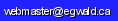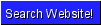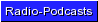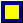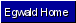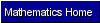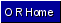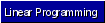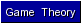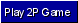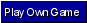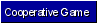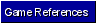Egwald Mathematics: Operations Research Linear Programming and Game Theory by Elmer G. Wiens Egwald's popular web pages are provided without cost to users. Please show your support by joining Egwald Web Services as a Facebook Fan:Follow Elmer Wiens on Twitter:A. Operations Research - Linear Programming 1. The linear programming home page. 2. Step through a linear programming graphical example. Then solve a problem by hand using the simplex algorithm, or the dual simplex algorithm. 3. Use either the primal simplex tableaux generator, or the dual simplex tableaux generator, to set up and solve your own linear programming problem, with a user-friendly interface. 4. Perform a sensitivity and/or postoptimal analysis of the solution to the data of an economics application of linear programming, with a user-friendly interface.             a. The profit maximization decision for a firm.             b. The least cost formula decision for a firm. 5. Step through a linear programming example using the online linear programming solver. 6. Use the online linear programming solver to solve your own L. P. problem. 7. Try your skill on these sample problems. 8. A few linear programming references. 9. Practical use of linear programming: linear programming is used in the oligopoly / public firm model to derive the bonus rates for the managers of a public firm. See the derivation of the oligopoly/public firm model. 10. A good back ground in linear algebra helps one to understand the concepts behind linear programming. B. Operations Research - Game Theory 1. Learn the terms and concepts behind game theory in the introduction to game theory. 2. Play a zero sum two person game online. 3. Create and play online your own zero sum two person game. 4. Use the online linear programming solver to solve a zero sum two person game. 5. Learn key concepts from the theory of cooperative, two person non-zero-sum games by stepping through the solution of the "Battle of the Sexes," based on the pioneering work of John Nash. 6. Discover the inescapable dilemma in the Prisoner's Dilemma - a noncooperative, two person non-zero-sum game. 7. What is the free-rider problem? How does it inhibit group rationality? 8. Analyze the Game of Chicken from the James Dean movie, Rebel Without a Cause. 9. The decisions of players in a game where players move sequentially, one after the other, can be modelled with von Neumann and Morgenstern's metagame. The characters in Hermann Melville's short story, Billy Budd, can be viewed as players in a sequence of interdependent, overlapping games, where the events transmitted in the text follow the metagame equilibria of the games. 10. For more about non-zero sum two person games see Mike Shor's Game Theory .net at Vanderbilt University. 11. Resource Libraries for Game Theory:       Eric Weisstein's World of Mathematics game theory pages are a great resource library for concepts used in games.       Books and links to game theory sites - Geometry.net - the online learning center. C. More Operations Research Sites on the Web. 1. The Math Forum Internet Mathematics Library has an extensive list of sites for operations research. http://mathforum.org/library/topics/operations_research/. Return to egwald.ca.Copyright © Elmer G. Wiens:   Egwald Web ServicesAll Rights Reserved.    Inquiries